# High School Physics : Identifying Forces and Systems

## Example Questions

### Example Question #1 : Identifying Forces And Systems

You are accelerating your car down a straight, level road.  Which free body diagram represent the forces on the car?  Neglect the effect of air resistance.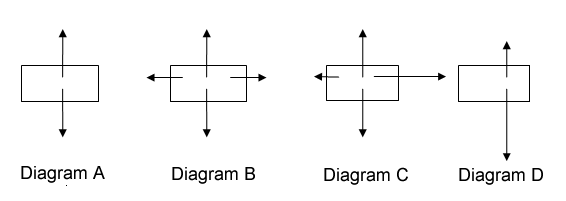Possible Answers:

Diagram C

Diagram D

Diagram A

Diagram B

Correct answer:

Diagram C

Explanation:

Begin by identifying what forces are acting on the car.  To start, the car has weight (the gravitational force) acting on it.  This force points down.  The car also has a normal force acting on it keeping it from falling through the road.  This force points upward and is equal in magnitude (size) to the weight force.  The car is accelerating, in the horizontal direction, which means that the forces must be unbalanced.  If the forces were balanced in the horizontal direction, the car would either not be moving or moving at a constant velocity.  The car likely is also undergoing friction which would be less than the accelerating force.  Therefore diagram C is correct as there is an unbalanced force in the horizontal direction with a friction force opposing the motion.

### Example Question #2 : Identifying Forces And Systems

A physics book is resting on a desk.  You push horizontally (from the side) on the book and the book moves across the desk at a constant speed.  Which free body diagram represents the force on the physics book?  Neglect the effect of air resistance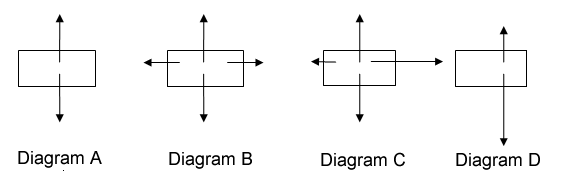Possible Answers:

Diagram D

Diagram C

Diagram A

Diagram B

Correct answer:

Diagram B

Explanation:

Begin by identifying the forces on the book.  The book has a weight (gravitational force) pushing down on it.  The book is sitting on a desk, therefore the desk has an upward normal force acting on the book keeping it from falling through the desk which is equal in magnitude (size) to the gravitational force.  The book is being pushed from the side meaning there are horizontal forces at work.  The first is an applied force, the second is a force of friction against the motion.  Since the book is moving at a constant velocity, the book is not accelerating.  This means that the forces must be balanced.  Therefore Diagram B is the correct answer as the horizontal forces are balanced.

### Example Question #3 : Identifying Forces And Systems

A baseball is hit with a bat.  While the ball flies through the air, which forces act on the ball?  Neglect air resistance.

Possible Answers:

The force of the bat acting on the ball

The force of gravity acting on the ball

The force of the ball moving forward through the air

All of the above

Both the force of gravity and the force of the ball moving forward through the air

Correct answer:

The force of gravity acting on the ball

Explanation:

Once the ball leaves the bat, there is no longer a contact force applied on the ball in the upward direction.  There are no horizontal forces at work either.  The only force acting on the ball is gravity.  Gravity points in the downward direction.

### Example Question #4 : Identifying Forces And Systems

A man is painting a house. He notices that there is a small drop of paint that is remaining perfectly still on the vertical wall. What conclusion can he draw about the paint?

Possible Answers:

The force of friction on the drop is equal to the force of gravity on it

We need to know the mass of the drop to draw any conclusions

The paint is water based

The drop of paint is very close to the ground, thus giving it a low potential energy

We need to know the density of the drop to draw any conclusions

Correct answer:

The force of friction on the drop is equal to the force of gravity on it

Explanation:

If the drop is at rest, then that means that the net forces acting upon it are equal to zero. There are two forces acting on the drop: the force due to gravity and the frictional force of the paint on the wall.

Mathematically, we can set up an equation for the net force: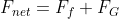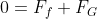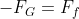The forces of friction and gravity are going to be equal and opposite, causing the drop to remain still on the wall.

### Example Question #5 : Identifying Forces And Systems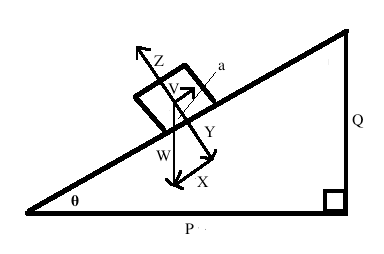Based on the diagram, which of the following statements is not true?

Possible Answers: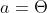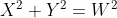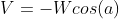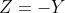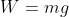Correct answer:Explanation:

In this diagram,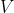represents the force due to friction. The equation for the force due to friction is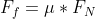, where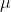is the coefficient of friction.

In this case,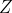represents the normal force. We can re-write the equation for friction: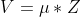can be re-written in terms of the angle, but will always need to be multiplied by the coefficient of friction in order to give an equation for.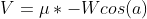The other equations are true.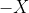and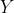form a right angle, so the Pythagorean theorem applies.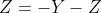is the normal force, which is, by definition, equal and opposite the vertical force of gravity.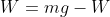is the total force of gravity, which will be equal to the mass times the acceleration of gravity.- the triangle formed by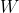,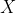, andis similar to the triangle formed by the surface,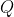, and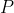, meaning that these angles must be equal.

### Example Question #1 : Identifying Forces And SystemsHow can we use this diagram to help calculate the net force on the object?

Possible Answers: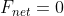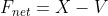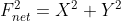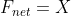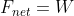Correct answer:Explanation:

For this problem, we need to break the forces into horizontal and vertical components. The only difference with an inclined plane is that you have to translate the horizontal plane to be parallel to the surface upon which the object is traveling, and the vertical plane to be perpendicular to the surface upon which the object is traveling.

Luckily, this is already done in the diagram, with the force of gravity () broken into the vertical component () and horizontal component ().

Now, we need to sum the forces in each plane. The forces in the vertical plane will be perpendicular to the surface:and.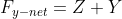Sinceandare opposite and equal forces: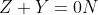There is no net force in the vertical plane. This makes sense, as we would not expect the object to have any movement perpendicular to the surface on which it is sliding.

Now we can sum the horizontal forces:and.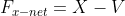Note thatis positive and V is negative. This is becauserepresents the force of friction, and is opposite to the force of.

Since there is no force in the vertical plane, this gives our final net force:### Example Question #7 : Identifying Forces And SystemsIf the mass of the object is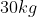and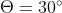, what is the normal force on the object?  Assume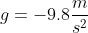Possible Answers: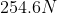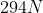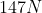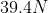Correct answer:Explanation:

The normal force is always perpendicular to the surface upon which the object is moving, and is pointed away from said surface. That means we are looking for the value forin the diagram.

Observe thatandare equal, but opposite forces.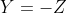If we can solve for, then we can find.

We can use our understanding of trigonometry to find an equation for.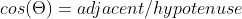If we plug in a for the angle, we see: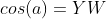Since we are solving for Y, we can multiply both sides by W.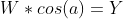Now that we know an equation for, we can return to our original equation to solve for.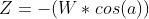From here, we can use Newton's second law to find the value of, the total force of gravity.Substitute this into our equation for.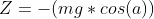Now we can solve forusing the values given in the question for the angle, mass, and gravity.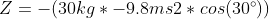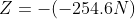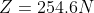### Example Question #8 : Identifying Forces And Systems

A ball is throwing and is moving upward through the air.  Which free body diagram represents the force on the ball?  Neglect the effect of air resistance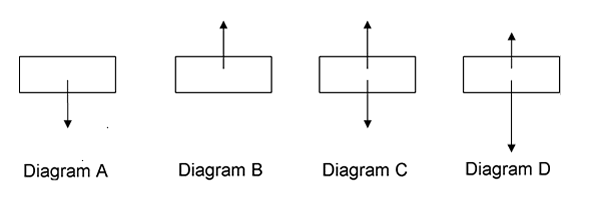Possible Answers:

Diagram C

Diagram A

Diagram B

Diagram D

Correct answer:

Diagram A

Explanation:

Once the ball leaves your hand, there is no longer a contact force applied on the ball in the upward direction.  There are no horizontal forces at work either.  The only force acting on the ball is gravity.  Gravity points in the downward direction.  Therefore Diagram A is the correct answer.

### Example Question #9 : Identifying Forces And Systems

An object moves forward with a constant velocity. What additional information do we need to know to determine the force acting upon the object?

Possible Answers:

The time the object is in motion

The mass of the object

The force is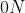The distance the object travels

The velocity of the object

Correct answer:

The force isExplanation:

Force is given by the product of mass and acceleration. If an object has a constant velocity, then it has no acceleration.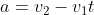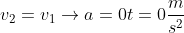If an object has no acceleration, then it must also have no net force.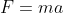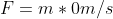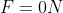No additional information is needed to solve this question.

### All High School Physics Resources